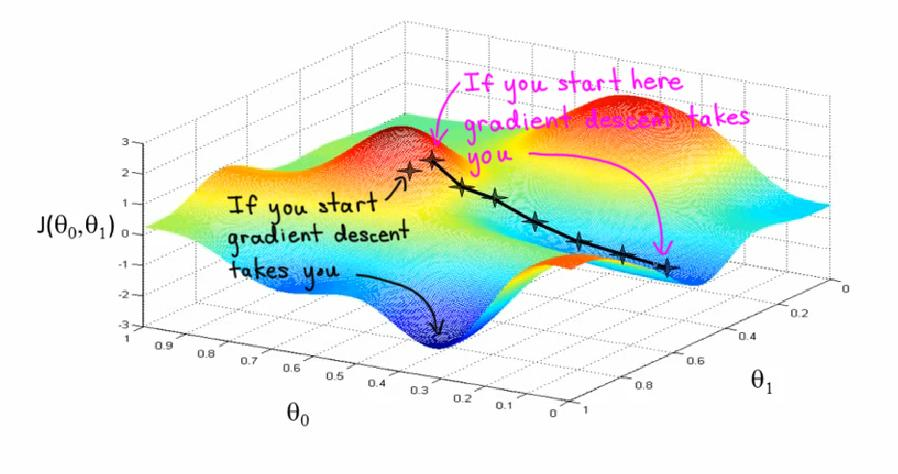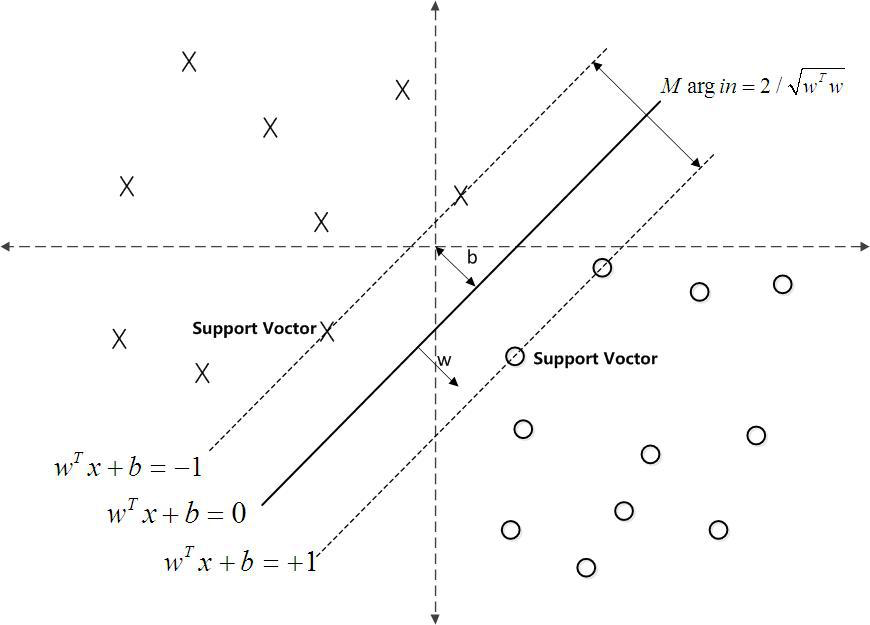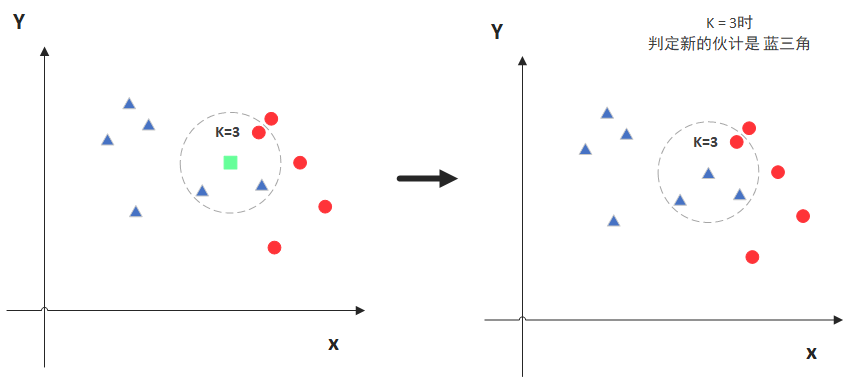# 机器学习中的核心算法 分类器算法

Posted by R1NG on August 10, 2021 Viewed Times

# 机器学习中的核心算法: 分类器算法

## 1. 随机梯度分类器梯度分类器 就是其 判别函数 在训练过程中使用了梯度下降法进行最优化的分类器.

批量梯度下降法 在每一次迭代中都会使用整个训练数据集作为其样本, 要计算数据集中所有样本的训练误差, 因此在数据集庞大时训练速度明显不如人意, 但可以确保在每次迭代中都顾及全体样本, 迭代方向一定和整体最优一致.

小批量梯度下降法 的迭代策略是前二者的混合, 其训练速度和模型精度介于二者之间. 它将整个训练数据集分为数组, 在每一次迭代时 随机抽取一组, 并计算这一组中所有样本的训练误差. 这样虽然无法确保迭代方向一定和整体最优一致, 但在大幅缩短训练耗时的同时也明显减小了迭代方向偏离整体最优的概率.

随机梯度分类器 就是其 判别函数 在训练过程中使用了随机梯度下降法进行最优化的分类器.

## 2. 线性支持向量机分类器

线性支持向量机支持向量机 的一个子类. 将数据集中的参数投影到某一个平面或空间 (这取决于参数的维度) 上, 若只需要使用一条直线, 平面或超平面即可将属于两种类别的参数完全隔离开, 我们就称这样的数据集是 线性可分 的, 而用于区分 线性可分 数据集的支持向量机分类器就被称为 线性支持向量机分类器.

$w \cdot x + b = 0$

$f(x_i) = \text{sign}(w \cdot x_i + b)$

$$w \cdot x + b = 0$$ 其中 $w : (_1, w_2, \cdots, w_n)$, 空间上某点 $x: (x_1, x_2, \cdots, x_n)$ 到该平面的 几何距离

$d = \frac{w_1 \cdot x_1 + w_2 \cdot x_2 + \cdots + w_n \cdot x_n + b}{\sqrt{w_1^2 + w_2^2 + \cdots + w_n^2}} = \frac{\vert w \cdot x + b\vert}{\vert \vert w \vert \vert}.$

$\frac{\vert w \cdot x + b\vert}{\vert \vert w \vert \vert}$

$-\frac{\vert w \cdot x + b\vert}{\vert \vert w \vert \vert}$

$y_i \cdot \frac{\vert w \cdot x + b\vert}{\vert \vert w \vert \vert}$

$-y_i \cdot \frac{\vert w \cdot x + b\vert}{\vert \vert w \vert \vert}$

$\text{L}(w, b) = - \sum_{i=1}^{n} y_i \cdot (w \cdot x_i + b)$

$w * x + b = 0.$$d_i = \frac{\vert w \cdot x_i + b\vert}{\vert \vert w \vert \vert}$

$D = \text{min}(d_i), i \in [n]$

$\begin{cases} \text{max}(D) \\ \frac{\vert w \cdot x_i + b\vert}{\vert \vert w \vert \vert} \geqslant D, i \in [n] \end{cases}$

## 3. 朴素贝叶斯分类器

1. 将训练数据集视作一个分类的类别和总数已知的待分类项集合.
2. 分别计算统计训练数据集中元素的特征属性 $a_1, a_2, \cdots, a_m$ 在各类别 $y_1, y_2, \cdots, y_n$ 下的 $m \cdot n$ 个条件概率估计 $$P(a_i \vert y_j), ~~ i \in [m], ~ j \in [n].$$
3. 基于特征属性条件独立的假设, 计算 $$P(y_i \vert x) = \frac{P(y_i) \prod_{j=1}^{m}P(a_j \vert y_i)}{P(x)} = \frac{P(x \vert y_i)P(y_i)}{P(x)}.$$

## 4. K 近邻分类器

K 近邻算法又称 K Nearest Neighbors, 顾名思义就是一个 “和临近数据值” 有关的算法.KNN 是一个 惰性算法, 这意味着它的全部计算过程只在对新的数据点进行分类时发生. 而基于此特征和其工作原理我们显然可以得知, KNN 在被应用到较大的数据集时需要更多的计算时间, 因为在分类新数据点时程序需要计算出新数据点到训练数据集中每一个数据点的距离, 将其排序并取前 K 个最小的, 这意味着对存储资源和计算资源的双重消耗.

1. KNN 是一个无需参数, 无需假设, 不需要事先对数据进行大量训练明显消耗时间的算法模型.
2. KNN 原理简单, 便于实现和使用, 但是不适用于规模庞大的数据集.
3. K 值会显著影响算法的分类表现, 在实践中我们常使用 交叉分类 获取最佳的 K 值.
4. 使用 KNN 算法所构造的分类器就被称为 K 近邻分类器.